# MA.912.NSO.1.2Export Print
Generate equivalent algebraic expressions using the properties of exponents.

### Examples

The expression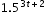is equivalent to the expression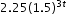which is equivalent to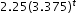.
General Information
Subject Area: Mathematics (B.E.S.T.)
Strand: Number Sense and Operations
Status: State Board Approved

## Benchmark Instructional Guide

• Base
• Expression
• Exponent

### Vertical Alignment

Previous Benchmarks

Next Benchmarks

### Purpose and Instructional Strategies

In grade 8, students generated equivalent algebraic expressions using the Laws of Exponents with integer exponents. In Algebra I, students expand this work to include rational-number exponents. In later courses, students extend the Laws of Exponents to algebraic expressions with logarithms.
• Instruction includes using the terms Laws of Exponents and properties of exponents interchangeably.
• Instruction includes student discovery of the patterns and the connection to mathematical operations (MTR.5.1).
• Students should be able to fluently apply the Laws of Exponents in both directions.
• For example, students should recognize that $a$is the quantity ($a$3)2; this may helpful when students are factoring a difference of squares.
• When generating equivalent expressions, students should be encouraged to approach from different entry points and discuss how they are different but equivalent strategies (MTR.2.1).
• The expectation for this benchmark does not include the conversion of an algebraic expression from exponential form to radical form and from radical form to exponential form.

### Common Misconceptions or Errors

• Students may not understand the difference between an expression and an equation.
• Students may not have fully mastered the Laws of Exponents and understand the mathematical connections between the bases and the exponents.
• Student may believe that with the introduction of variables, the properties of exponents differ from numerical expressions.

### Strategies to Support Tiered Instruction

• Instruction includes the opportunity to distinguish between an expression and an equation. These should be captured in a math journal.
• For example, when generating equivalent expressions, place an equal sign in between the expressions and label each expression and the equation.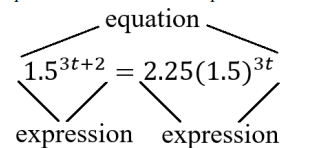• Instruction provides opportunities to write each term in expanded form first and then use Laws of Exponents to combine like factors. It may also be helpful to chunk each step.
• For example, to rewrite the expression (8$x$3)with one exponent, write out (8)($x$)($x$)($x$)(8)($x$)($x$)($x$) and then use the commutative property to write (8)(8)($x$)($x$)($x$)($x$)($x$)($x$) = 64$x$6
• Teacher provides instruction for problems that require multiple applications of the Laws of Exponents by chunking the steps so that students are applying one property at each time and explaining the property applied. Each time ask students to identify the property of exponents that they applied.
• Teacher provides students side-by-side problems, one with variable bases and the other choosing a value for the variable. As students work through the problems, ask them about the similarities in the problem-solving process.
• For example, teacher can model generating equivalent expressions like the ones below.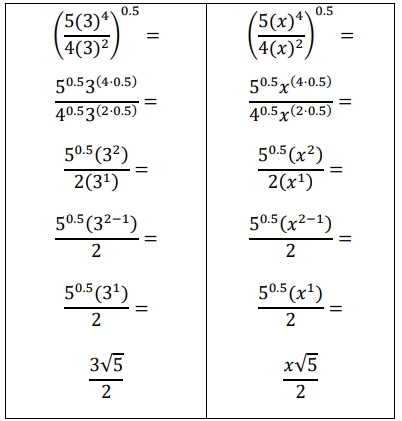• Teacher provides a review of the relationship between the base and the exponent by modeling an example of operations using a base and exponent.
• For example, determine the numerical value of 63.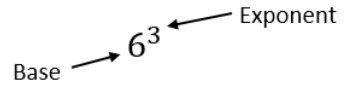• 63 which is equivalent to 6 ⋅ 6 ⋅ 6 which is equivalent to 216.

• Given the function $h$($x$) = 100.2$x$ , what is the rate of growth or decay?
• Instructional Task 2 (MTR.3.1, MTR.4.1
• Part A. Write the algebraic expression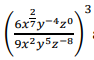as an equivalent expression where each variable only appears once.
• Part B. Compare your method of simplifying with a partner.

### Instructional Items

• Instructional Item 1
• Given the algebraic expression 2.32$t$−1, create an equivalent expression.
• Instructional Item 2
• Use the properties of exponents to create an equivalent expression for the given expression shown below with no variables in the denominator.
(64$x$²)$\frac{\text{1}}{\text{6}}$(32$x$5)$\frac{\text{2}}{\text{5}}$

*The strategies, tasks and items included in the B1G-M are examples and should not be considered comprehensive.

## Related Courses

This benchmark is part of these courses.
1200310: Algebra 1 (Specifically in versions: 2014 - 2015, 2015 - 2022, 2022 and beyond (current))
1200320: Algebra 1 Honors (Specifically in versions: 2014 - 2015, 2015 - 2022, 2022 and beyond (current))
1200380: Algebra 1-B (Specifically in versions: 2014 - 2015, 2015 - 2022, 2022 and beyond (current))
1200400: Foundational Skills in Mathematics 9-12 (Specifically in versions: 2014 - 2015, 2015 - 2022, 2022 and beyond (current))
7912090: Access Algebra 1B (Specifically in versions: 2014 - 2015, 2015 - 2018, 2018 - 2019, 2019 - 2022, 2022 and beyond (current))
1200315: Algebra 1 for Credit Recovery (Specifically in versions: 2014 - 2015, 2015 - 2022, 2022 and beyond (current))
1200385: Algebra 1-B for Credit Recovery (Specifically in versions: 2014 - 2015, 2015 - 2022, 2022 and beyond (current))
7912075: Access Algebra 1 (Specifically in versions: 2014 - 2015, 2015 - 2018, 2018 - 2019, 2019 - 2022, 2022 and beyond (current))
1200388: Mathematics for Data and Financial Literacy Honors (Specifically in versions: 2022 and beyond (current))
1200384: Mathematics for Data and Financial Literacy (Specifically in versions: 2022 and beyond (current))
7912120: Access Mathematics for Data and Financial Literacy (Specifically in versions: 2022 - 2023, 2023 and beyond (current))
1200710: Mathematics for College Algebra (Specifically in versions: 2022 and beyond (current))

## Related Access Points

Alternate version of this benchmark for students with significant cognitive disabilities.
MA.912.NSO.1.AP.2: Identify equivalent algebraic expressions using properties of exponents.

## Related Resources

Vetted resources educators can use to teach the concepts and skills in this benchmark.

## Formative Assessments

Roots and Exponents:

Students are asked to rewrite the square root of five in exponential form and justify their choice of exponent.

Type: Formative Assessment

Rational Exponents and Roots:

Students asked to show that two forms of an expression (exponential and radical) are equivalent.

Type: Formative Assessment

Rational Exponents - 4:

Students are asked to rewrite expressions involving radicals and rational exponents in equivalent forms.

Type: Formative Assessment

Rational Exponents - 2:

Students are asked to convert numerical expressions from exponential to radical form.

Type: Formative Assessment

Rational Exponents - 3:

Students are asked to convert a product of a radical and exponential expression to a single power of two.

Type: Formative Assessment

Rational Exponents - 1:

Students are asked to convert numerical expressions from radical to exponential form.

Type: Formative Assessment

College Costs:

Students are asked to transform an exponential expression so that the rate of change corresponds to a different time interval.

Type: Formative Assessment

Population Drop:

Students are asked to use the properties of exponents to show that two expressions are equivalent and compare the two functions in terms of what each reveals.

Type: Formative Assessment

## MFAS Formative Assessments

College Costs:

Students are asked to transform an exponential expression so that the rate of change corresponds to a different time interval.

Population Drop:

Students are asked to use the properties of exponents to show that two expressions are equivalent and compare the two functions in terms of what each reveals.

Rational Exponents - 1:

Students are asked to convert numerical expressions from radical to exponential form.

Rational Exponents - 2:

Students are asked to convert numerical expressions from exponential to radical form.

Rational Exponents - 3:

Students are asked to convert a product of a radical and exponential expression to a single power of two.

Rational Exponents - 4:

Students are asked to rewrite expressions involving radicals and rational exponents in equivalent forms.

Rational Exponents and Roots:

Students asked to show that two forms of an expression (exponential and radical) are equivalent.

Roots and Exponents:

Students are asked to rewrite the square root of five in exponential form and justify their choice of exponent.

## Student Resources

Vetted resources students can use to learn the concepts and skills in this benchmark.

## Parent Resources

Vetted resources caregivers can use to help students learn the concepts and skills in this benchmark.